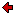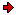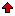Journal of Technical
Physics

A QUARTERLY JOURNALPrevious issue vol. 45, no. 4 (2004) vol. 46, no. 1 (2005)Next issuevol. 46, no. 2 (2005)

### Contents of issue 1, vol. 46

1. S. Piekarski: Stress-assisted diffusion and the modified Fick law
2. A. Trzęsowski: Tensility and compressibility of axially symmetric nanoclusters II: Cylindrical nanoclusters
3. F.D. Zaman, K. Masood: Direct and inverse acoustic scattering problem over a two-part impedance ground in a moving fluid by using Wiener-Hopf technique
4. Z. Koruba: A mathematical model of the dynamics and control of a gyroscopic platform mounted on board of an aerial vehicle
Brief Notes
1. T. Lenkowska-Czerwińska: Vortices in the inhomogeneous superconducting states of high-Tc superconductivity

S. Piekarski: Stress-assisted diffusion and the modified Fick law
It can be observed that the equation for "stress-assisted diffusion" applied by Shewmon (P.G. Shewmon, Diffusion in solids, McGraw-Hill, 1963) is simply the Einstein-Smoluchowski equation.
In turn, for a constant temperature field, the modified Fick law (introduced in: S. Piekarski, On the modified Fick law and its potential applications, J. Tech. Phys., 44, 2, 125-131, 2003) reduces to the Einstein-Smoluchowski equation.
Therefore, the solutions of the modified Fick law can be adopted to the problems considered by Shewmon. In this paper, the problems of diffusion and thermodiffusion in the strain fields of dislocations (screw and edge) are considered and new solutions are briefly discussed.

ContentsA. Trzęsowski: Tensility and compressibility of axially symmetric nanoclusters II: Cylindrical nanoclusters
A method to model the tensile properties of cylindrical nanoclusters is proposed. The isothermal size effect, appearing in the static simple tension test and in the dynamic process of uniaxial stretching, is discussed. A numerical simulation of this effect is presented.

ContentsF.D. Zaman, K. Masood: Direct and inverse acoustic scattering problem over a two-part impedance ground in a moving fluid by using Wiener-Hopf technique
The aim of this paper is to solve the direct and inverse problem in a moving fluid. We consider the direct and inverse scattering problem of acoustic line source by a two-part boundary of a half-space, having a small variation in propagation speed in the presence of a moving fluid. The problem reduces to the solution of two integral equations by using the Fourier transform and Green's function. One of these equations is solved exactly by the Wiener-Hopf technique while the other is reduced to a Fredholm equation of the first kind whose kernel involves the solution to the first equation. The procedure can be applied to recover the variation in the wave speed over a nonhomogeneous impedance ground.

ContentsZ. Koruba: A mathematical model of the dynamics and control of a gyroscopic platform mounted on board of an aerial vehicle
The work deals with simplified mathematical models of uni-, bi-, and triaxial gyroscopic platforms mounted on board of an aerial vehicle. The platforms can be used as a reference of the head for detecting, tracing and laser illuminating of a ground target or as the base of a television or thermovision camera. The models permit monitoring the platforms, controlling and correcting them, if affected by external disturbances and kinematic interaction with the base (i.e. board of the aerial vehicle).

ContentsBrief Notes
T. Lenkowska-Czerwińska: Vortices in the inhomogeneous superconducting states of high-Tc superconductivity
We investigate the Josephson effect in the stripe phase of in-plane superconductivity of the layered materials. The Ginzburg-Landau model of the mixed in-plane state, s+id, is formulated and the characteristic length scales for the Josephson effect are discussed.
The 3D Lawrence-Doniach type model of the stripe state is constructed. The system of equations for the phase of the order parameter is obtained in the London-type approximation. The structure of the pancake and Josephson vortices in the weakly coupled stripes is analyzed.

ContentsReprints of the full papers may be obtained from their authors. Contact Editorial Office in case you need the address of the respective author.
 Home Editors Scope Abstracts For authors Subscription